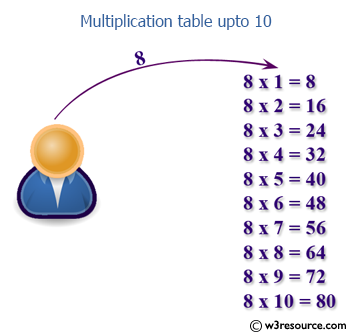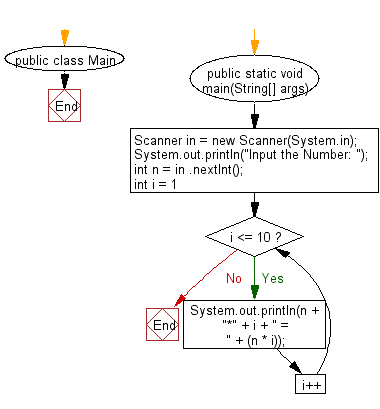﻿ Java exercises: Print multiplication table of a number upto 10 - w3resource# Java Exercises: Print multiplication table of a number upto 10

## Java Basic: Exercise-7 with Solution

Write a Java program that takes a number as input and prints its multiplication table upto 10.

Test Data:
Input a number: 8

Pictorial Presentation:Sample Solution:

Java Code:

``````import java.util.Scanner;

public class Exercise7 {

public static void main(String[] args) {
Scanner in = new Scanner(System.in);

System.out.print("Input a number: ");
int num1 = in.nextInt();

for (int i=0; i< 10; i++){
System.out.println(num1 + " x " + (i+1) + " = " +
(num1 * (i+1)));
}
}
}
```
```

Sample Output:

```Input a number: 8
8 x 1 = 8
8 x 2 = 16
8 x 3 = 24
8 x 4 = 32
8 x 5 = 40
8 x 6 = 48
8 x 7 = 56
8 x 8 = 64
8 x 9 = 72
8 x 10 = 80
```

Flowchart:Sample Solution:

Java Code:

``````import java.util.Scanner;

public class Main {

public static void main(String[] args) {
Scanner in = new Scanner(System.in);
System.out.println("Input the Number: ");
int n = in .nextInt();
for (int i = 1; i <= 10; i++) {
System.out.println(n + "*" + i + " = " + (n * i));
}
}
}
```
```

Sample Output:

```Input the Number:
6
6*1 = 6
6*2 = 12
6*3 = 18
6*4 = 24
6*5 = 30
6*6 = 36
6*7 = 42
6*8 = 48
6*9 = 54
6*10 = 60
```

Flowchart:Java Code Editor:

What is the difficulty level of this exercise?

Test your Programming skills with w3resource's quiz.

﻿

## Java: Tips of the Day

countOccurrences

Counts the occurrences of a value in an array.

Use Arrays.stream().filter().count() to count total number of values that equals the specified value.

```public static long countOccurrences(int[] numbers, int value) {
return Arrays.stream(numbers)
.filter(number -> number == value)
.count();
}
```

Ref: https://bit.ly/3kCAgLb